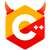# 3732. 纸条#include <algorithm>
#include <iostream>
#include <vector>
using namespace std;

int main() {
int n, m, k;
unsigned long long x;
string text;
cin >> n >> m >> k >> x >> text;
vector<string> dict(m);
for (int i = 0; i < m; ++i) {
cin >> dict[i];
sort(dict[i].begin(), dict[i].end());
}
vector<char> index(m);
--x;  // 注意 x 给的是「第几个」数，所以实际上的数字是 x - 1。
for (auto i = index.rbegin(); i != index.rend(); ++i) {
*i = x % k;
x /= k;
}
for (int i = 0, cnt = 0; cnt < m; ++i) {
if (text[i] == '#') {
text[i] = dict[cnt][index[cnt]];
++cnt;
}
}
cout << text << '\n';
}